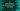# C program to find the maximum and minimum number in an array### C program to find out the maximum and minimum number in an array :

In this c programming tutorial, we will learn how to find the maximum and minimum number in an array using ‘c’. All the elements for the array, we will take as input from the user. Then, we will find out maximum and minimum number in the array using two different functions. I will explain in step by step but first take a look into the program :

### C Program :

``````#include

//6
int find_max(int arr[],int size){
int i;
//7
int max = -1;

//8
for(i=0;i<size;i++){ if(arr[i] > max){
max = arr[i];
}
}

//9
return max;
}

//10
int find_min(int arr[],int size){
int i;

//11
int min = arr;

//12
for(i=1;i<size;i++){
if(arr[i]<min){
min = arr[i];
}
}

//13
return min;
}

int main(){
//1
int i,total;

//2
printf("Enter total no of elements : ");
scanf("%d",&total);

//3
int myArray[total];

//4
for(i=0;i<total;i++){
printf("Enter no %d : ",i+1);
scanf("%d",&myArray[i]);
}

//5
int maximumNo = find_max(myArray,total);
int minimumNo = find_min(myArray,total);

//14
printf("Maximxum number in the array is %d \n",maximumNo);
printf("Minimum number in the array is %d \n",minimumNo);
}``````

### Explanation of the program :

The commented numbers in the above program indicates the below steps :

1. Create two variables i and total.
2. Take the size of the array as input from the user and save it in the variable total.
3. Create one new array myArray and the size of the array should be same as the variable total.
4. Use one for loop and read all elements . Store the elements in the array myArray.
5. Now, find the maximum and minimum numbers in the array using two different functions findmax_ and findmin_ . Save these values in the int variables maximumNo and minimumNo.
6. findmax_ method is defined as int findmax(int arr[],int size)_ i.e. it takes one array and its size as input and returns one int variable. This return value is the maximum value in the input array.
7. Create one integer variable max and store -1 in it. We are storing one minimum value in this variable so that we can compare it with other elements of the array and update the max value.
8. Now, run one for loop and check for each element in the array. If value of a element is greater than max, set its value to max.
9. After the for loop is completed, the max variable will hold the maximum value of the array. Return this variable from the function.
10. Similar to findmax_ , findmin_ finds the minimum value in an array . int findmin(int arr[],int size)_ finds the minimum value in the array arr.
11. The process is similar to the above function. First we have initialized one variable min and store the first element of the array in it.
12. We will start scanning the elements starting from the second element using one for loop. We will compare it with each element and if any element is less than min, set this value to min.
13. After the loop completes, return the min value.
14. Finally, print the maximum and minimum values.

### Example Outputs :

``````Enter total no of elements : 5
Enter no 1 : 34
Enter no 2 : 10
Enter no 3 : 23
Enter no 4 : 13
Enter no 5 : 99
Maximxum number in the array is 99
Minimum number in the array is 10

Enter total no of elements : 2
Enter no 1 : 2
Enter no 2 : 1
Maximxum number in the array is 2
Minimum number in the array is 1

Enter total no of elements : 10
Enter no 1 : 1
Enter no 2 : 2
Enter no 3 : 3
Enter no 4 : 4
Enter no 5 : 5
Enter no 6 : 6
Enter no 7 : 7
Enter no 8 : 8
Enter no 9 : 9
Enter no 10 : -10
Maximxum number in the array is 9
Minimum number in the array is -10``````

### Where is the color and why codevscolor ?

Long story short, I love paintings and I paint on weekends. We(me and my wife) have one Youtube channel. Don't forget to subscribe ❤️❤️🤩